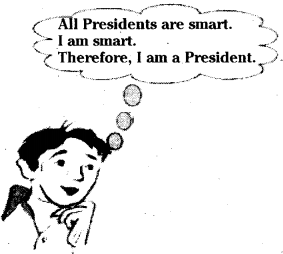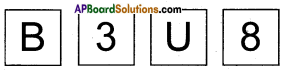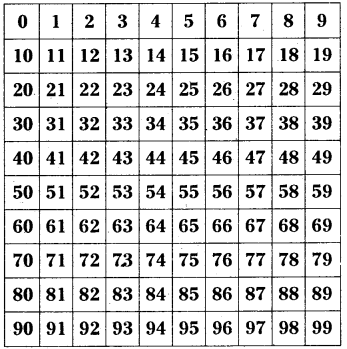# AP Board 9th Class Maths Solutions Chapter 15 Proofs in Mathematics Ex 15.2

AP State Syllabus AP Board 9th Class Maths Solutions Chapter 15 Proofs in Mathematics Ex 15.2 Textbook Questions and Answers.

## AP State Syllabus 9th Class Maths Solutions 15th Lesson Proofs in Mathematics Exercise 15.2Question 1.
Use deductive reasoning to answer the following.

i) Human beings are mortal. Jeevan is a human being. Based on these two statements, what can’ you conclude about Jeevan?
Solution:
From the above statements we can deduce that Jeevan is mortal as it is given that all humans are mortal and Jeevan is a human.

ii) All Telugu people are Indians. X is an Indian. Can you conclude that X belongs to Telugu people?
Solution:
No. X may belong to any other language like Tamil, Kannada, Malayali…. etc.

iii) Martians have red tongues. Gulag is a Martian. Based on these two statements, what can you conclude about Gulag?
Solution:

iv) What is the fallacy in the Raju’s reasoning in the cartoon belowSolution:
All smarts need not be a President. There could be some other persons who are smart too.

Question 2.
Once again you are given four cards. Each card has a number printed on one side and a letter on the other side. Which are the only two cards you need to turn .over to check whether the following rule holds ?
“If a card has a consonant on one side, then it has an odd number on the other side”.Solution:
You need to turn over B and 8 only. If B has an even number then the rule has broken. Similarly if 8 has a consonant on the other side then also the rule has been broken.

Question 3.
Think of this puzzle. What do you need to find a chosen number from this square ? Four of the clues below are true but do nothing to help in finding the number. Four of the clues are necessary for finding it.
Here are eight clues to use:
a) The number is greater than 9.
b) The number is not a multiple of 10.
c) The number is a multiple of 7.
d) The number is odd.
e) The number is not a multiple of 11.
f) The number is less than 200.
g) Its ones digit is larger than its tens digit.
h) Its tens digit is odd.
What is the number ?Solution:

 Hint Conclusion a The number may be from 10 to 99 (2nd clue implies, first clue is of no use) No use b The number is not any of (10, 20, 30, …………90) c The number may be any of (7, 14, 21,28, 35,42, …98) d The number may be any of (7,21,35,49, 63, 77,91) e The number may be any of (7, 21, 35, 49. 63. 91) f No use g The numbr may be 35, 49 h 35